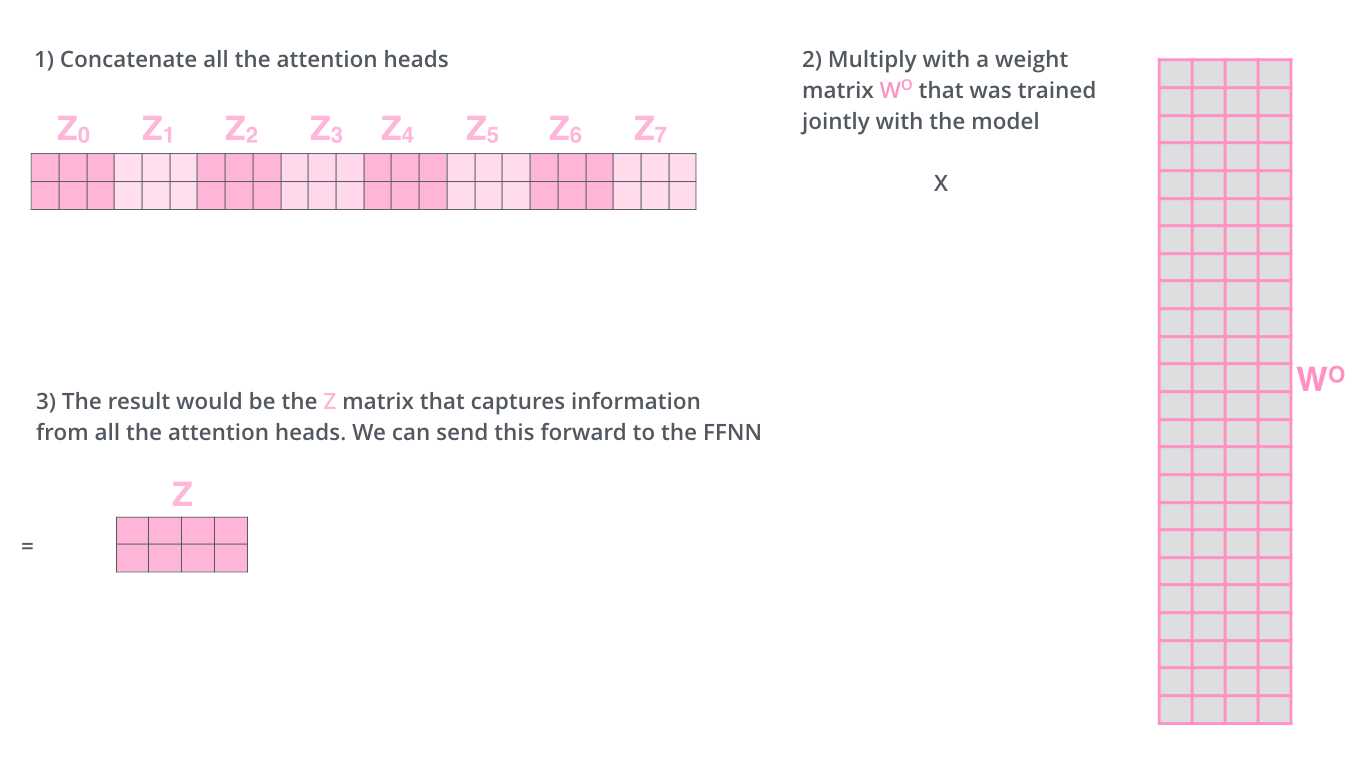## 原理介绍

### Self-attention 介绍

$$a = softmax(f(key, query))$$

$$c = \sum_{i=1}^{N}(a \times value)$$

self-attention 指的是 attention 得到的途径来源于编码器自身。假设有输入 X1 到 Xn，为了得到第 t 个输入对应的 attention，此时 query 就是 Xt（其实不是，还需要乘以一个权重W），K和V就是 X1 到 Xn（其实也不是，也要乘以一个权重）。

$$Attention (Q, K, V) = softmax(\frac{QK^T}{\sqrt{d_k}})V$$

$$head = Attention(QW_i^Q,KW_i^K,VW_i^V)$$## 实现过程

### 程序安装

（1）https://blog.csdn.net/weixin_42744102/article/details/87006081
（2）https://blog.csdn.net/weixin_42744102/article/details/87076089
（3）https://blog.csdn.net/weixin_42744102/article/details/87088748

### 化学反应数据收集与处理

Br C C O S ( = O ) ( = O ) C C -> Br C C O . Cl S ( = O) ( = O ) C C，由于括号、等号都有有特殊的含义，因此也都算作一个单词。最后把问题整理到一个文件，如 train_src，把回答整理到另一个文件，如train_tgt，就完成了数据的准备。